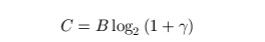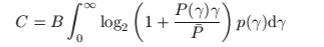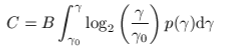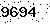FunWithElectronics.com- Collection of Information for those with Electronics as a Hobby
Up one level (Communication theory)

## Channel capacity

The channel capacity is given as the maximal rate one can use on a given channel. It is primarily dependent on the SNR ratio. For channels that vary in time, there are ways that a maximal capacity can be achieved depending on whether one have channel state information (CSI) at the receiver, at the transmitter and the receiver, or at none of them.

By using the word maximal rate, the absolute maximal rate if a perfect channel code is used is meant. It is impossible to make a code that performs better, and the capacity is always lower in practice than what can be achieved theoretically. There are however today codes that can achieve nearly the capacity.

The easiest capacity to calculate is the one for an AWGN channel (Additive white gaussian noise channel).

The channel capacity of a AWGN channel is:where gamma is the SNR

The channel capacity of an inverted fading channel is:where sigma is given by the SNR that one will have on the inverted channel:The channel capacity of a fading channel where variable power is used depending on channel quality is:With the optimal power allocation which is water filling, the channel capacity is given by:Fill in number